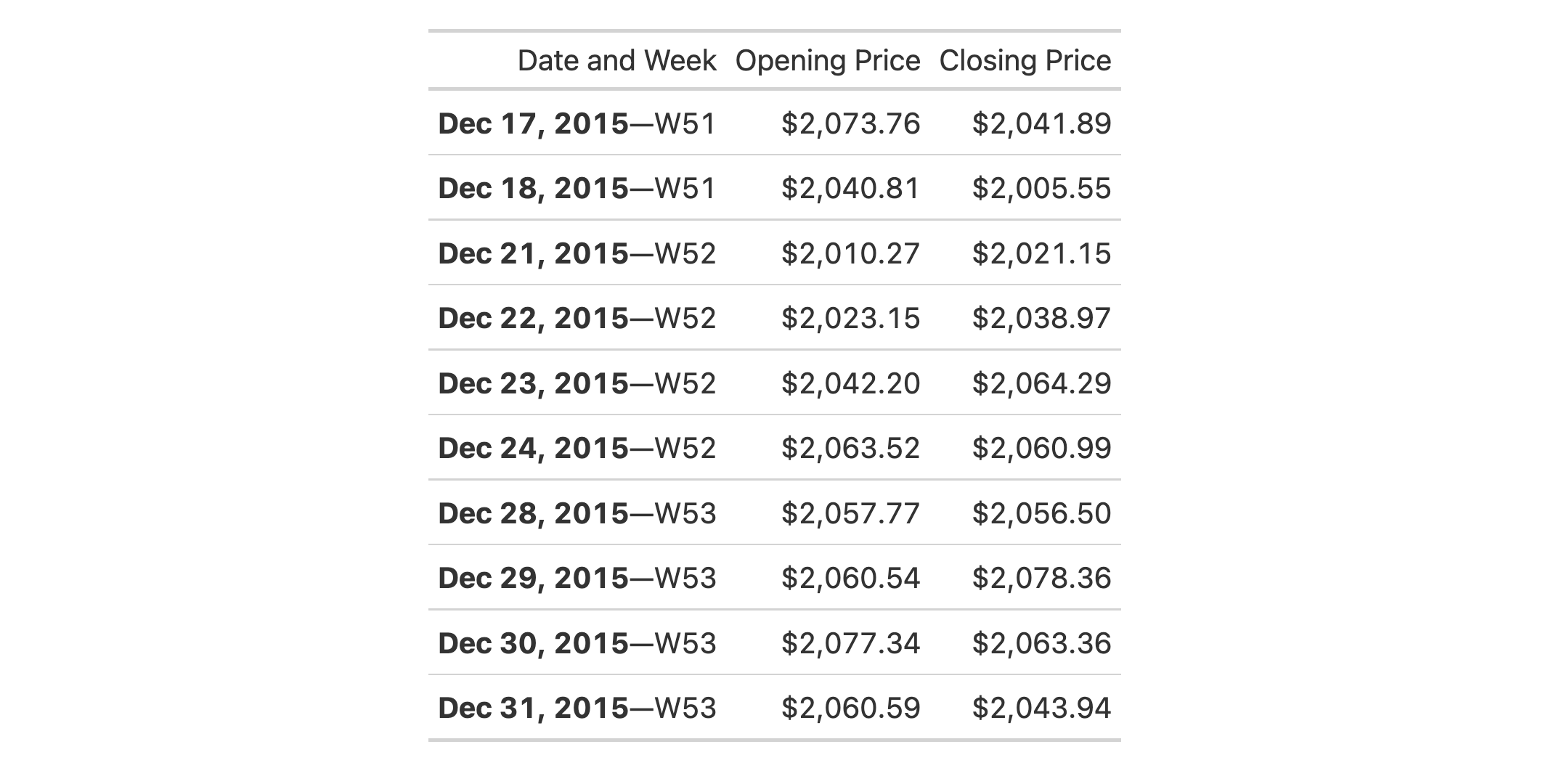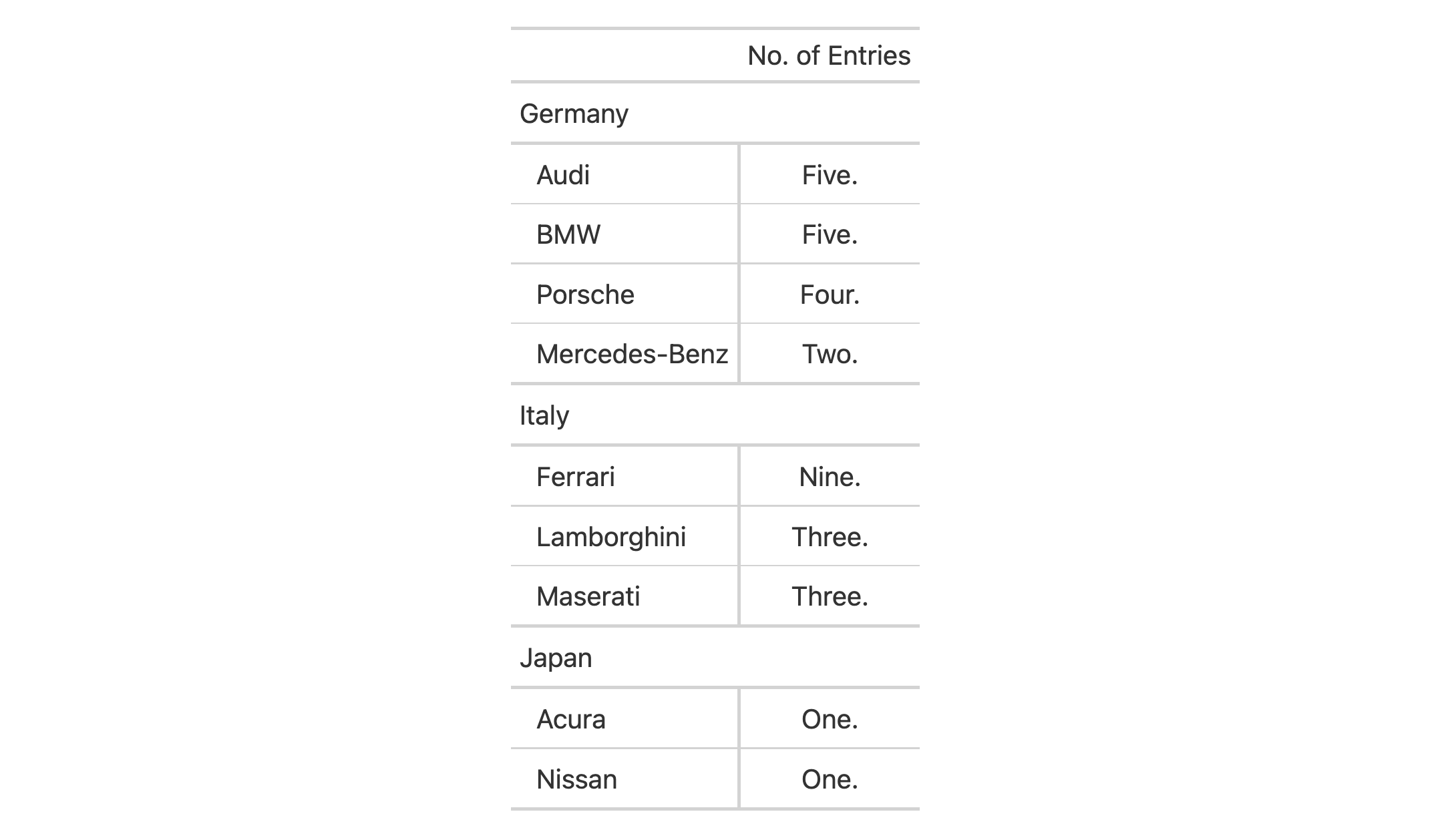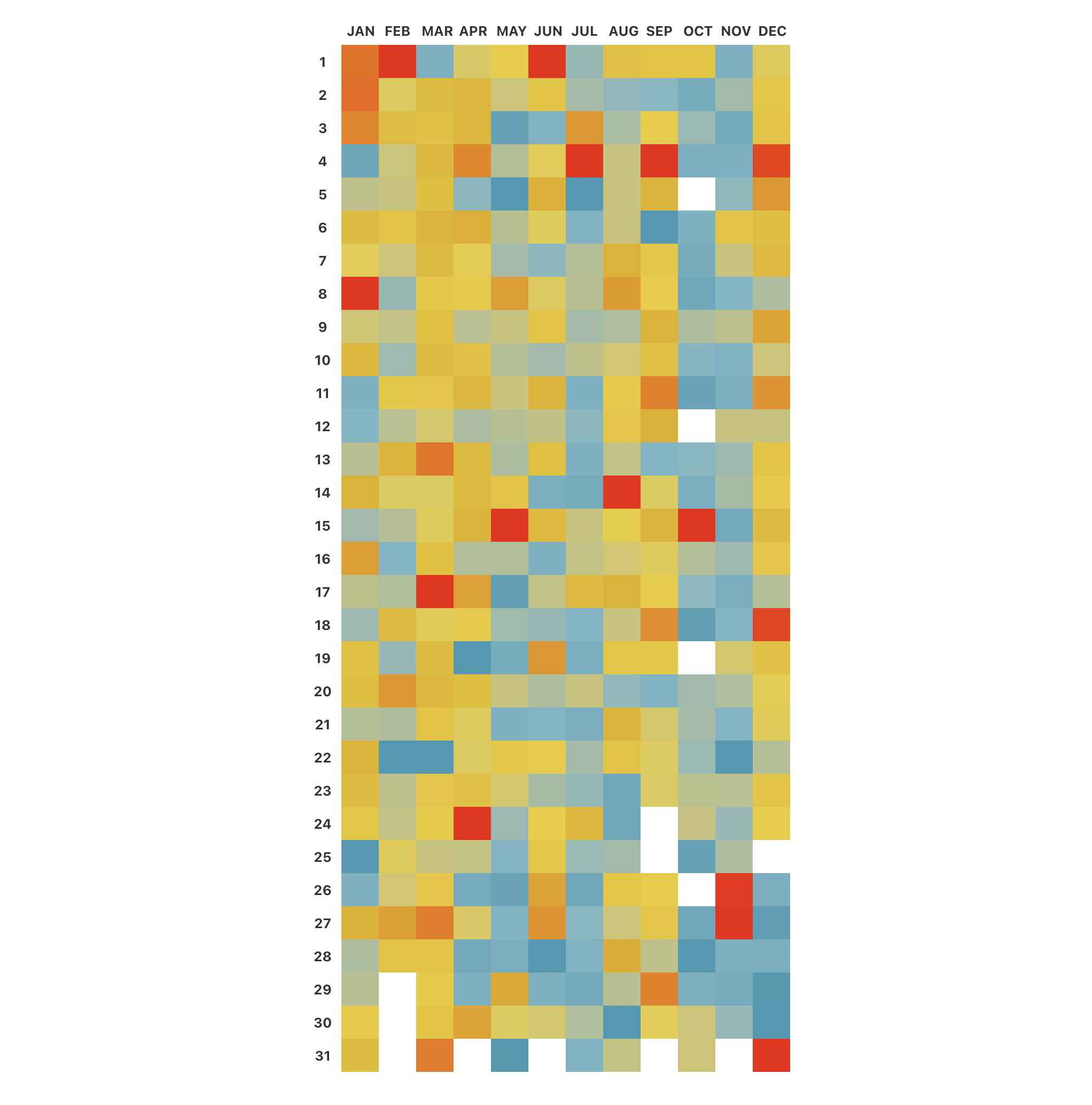Text transforming in gt is the act of modifying formatted strings in targeted cells. The text_transform() function provides the most flexibility of all the text_*() functions in their family of functions. With it, you target the cells to undergo modification in the locations argument while also supplying a function to the fn argument. The function given to fn should ideally at the very least take x as an input (it stands for the character vector that is essentially the targeted cells) and return a character vector of the same length as the input. Using the construction function(x) { .. } for the function is recommended.

## Usage

text_transform(data, fn, locations = cells_body())

## Arguments

data

The gt table data object

obj:<gt_tbl> // required

This is the gt table object that is commonly created through use of the gt() function.

fn

Function for text transformation

<function> // required

The function to use for text transformation. It should include x as an argument and return a character vector of the same length as the input x.

locations

Locations to target

<locations expressions> // default: cells_body()

The cell or set of cells to be associated with the text transformation. Only the cells_body(), cells_stub(), cells_row_groups(), cells_column_labels(), and cells_column_spanners() helper functions can be used here. We can enclose several of these calls within a list() if we wish to make the transformation happen at different locations.

## Value

An object of class gt_tbl.

## Examples

Use a subset of the sp500 dataset to create a gt table. Transform the text in the date column using a function supplied to text_transform() (via the fn argument). Note that the x in the fn = function (x) part consists entirely of ISO 8601 date strings (which are acceptable as input to the vec_fmt_date() and vec_fmt_datetime() functions).

sp500 |>
dplyr::select(date, open, close) |>
dplyr::arrange(-dplyr::row_number()) |>
gt() |>
fmt_currency() |>
text_transform(
fn = function(x) {
paste0(
"<strong>",
vec_fmt_date(x, date_style = "m_day_year"),
"</strong>",
"&mdash;W",
vec_fmt_datetime(x, format = "w")
)
},
locations = cells_body(columns = date)
) |>
cols_label(
date = "Date and Week",
open = "Opening Price",
close = "Closing Price"
)Let's use a summarized version of the gtcars dataset to create a gt table. First, the numeric values in the n column are formatted as spelled-out numbers with fmt_spelled_num(). The output values are indeed spelled out but exclusively with lowercase letters. We actually want these words to begin with a capital letter and end with a period. To make this possible, the text_transform() function will be used since it can modify already-formatted text. Through the fn argument, we provide a custom function that uses R's toTitleCase() operating on x (the numbers-as-text strings) within a paste0() so that a period can be properly placed.

gtcars |>
dplyr::select(mfr, ctry_origin) |>
dplyr::filter(ctry_origin %in% c("Germany", "Italy", "Japan")) |>
dplyr::group_by(mfr, ctry_origin) |>
dplyr::count() |>
dplyr::ungroup() |>
dplyr::arrange(ctry_origin, desc(n)) |>
gt(rowname_col = "mfr", groupname_col = "ctry_origin") |>
cols_label(n = "No. of Entries") |>
tab_stub_indent(rows = everything(), indent = 2) |>
cols_align(align = "center", columns = n) |>
fmt_spelled_num() |>
text_transform(
fn = function(x) {
paste0(tools::toTitleCase(x), ".")
},
locations = cells_body(columns = n)
)There may be occasions where you'd want to remove all text. Here in this example based on the pizzaplace dataset, we generate a gt table that summarizes an entire year of data by colorizing the daily sales revenue. Individual cell values are not needed here (since the encoding by color suffices), so, text_transform() is used to turn every value to an empty string: "".

pizzaplace |>
dplyr::group_by(date) |>
dplyr::summarize(rev = sum(price)) |>
dplyr::ungroup() |>
dplyr::mutate(
month = lubridate::month(date, label = TRUE),
day_num = lubridate::mday(date)
) |>
dplyr::select(-date) |>
tidyr::pivot_wider(names_from = month, values_from = rev) |>
gt(rowname_col = "day_num") |>
data_color(
method = "numeric",
palette = "wesanderson::Zissou1",
na_color = "white"
) |>
text_transform(
fn = function(x) "",
locations = cells_body()
) |>
opt_table_lines(extent = "none") |>
opt_all_caps() |>
cols_width(everything() ~ px(35)) |>
cols_align(align = "center")4-4

## Function Introduced

v0.2.0.5 (March 31, 2020)

Other text transforming functions: text_case_match(), text_case_when(), text_replace()